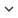ENGArticle
Article 519
Author/s
• B. Agbaogun, Jose M. Alonso, H. Buddenbaum, K. Fischer
DOI
Source
• Journal of Chemometrics, 2021 - Provisionally ranked Q1

# Modelling of the adsorption of urea herbicides by tropical soils with an Adaptive-Neural-based Fuzzy Inference System

Sorption of chemical compounds by soils holds a major consequence for their fate in the environment. As such, sorption coefficient (Kd/Koc) which is derived from laboratory or field experiments is a fundamental parameter used in almost all screening tools to evaluate the fate or mobility of environmentally relevant compounds in soils. This coefficient is controlled by many soil and solute specific properties, as well as environmental variables. Soft computing techniques such as Adaptive Neuro-Fuzzy Inference Systems (ANFIS) have been applied to predicting the equilibrium partitioning of many compounds in various engineered systems. Application of these techniques to natural systems such as soils is however lacking or very scanty. Here, we present the use of ANFIS in predicting the sorption capacity per unit mass of soil in the calculation of the sorption coefficient of compounds in soils. In a previous study we collected data associated to adsorption of five phenylurea herbicides in eighteen tropical soils. Here, we analyzed such data and based on established correlations, nine variables were selected as potential input vectors (i.e., six soil properties, two pesticide molecular descriptors, and initial solute concentrations). Then, a total of 255 ANFIS models of one to eight input vectors were elaborated under 10-fold cross-validation. The aim was finding out the smallest set of inputs ready to yield the most reliable prediction. Multiple linear regression (MLR) models were similarly developed, and compared with the ANFIS in terms of mean absolute error (MAE), root mean squared error (RMSE), and coefficient of determination (R2). The best ANFIS model (M94) has an MAEtest, RMSEtest and R2test of 3.43±0.43, 4.94±0.80 and 0.95±0.01 respectively; whereas the best MLR model (M13) returned an MAE, RMSE and R2 of 7.71±0.13, 10.11±1.21 and 0.81±0.01, respectively. We observed that generally ANFIS performed better than MLR regarding both accuracy and interpretability. Accordingly, we recommend the use of ANFIS for predicting sorption coefficients in soils.
Keywords: Sorption, phenylurea herbicides, tropical soils, modelling, Adaptive Neuro-Fuzzy Inference System, Multiple Linear Regression